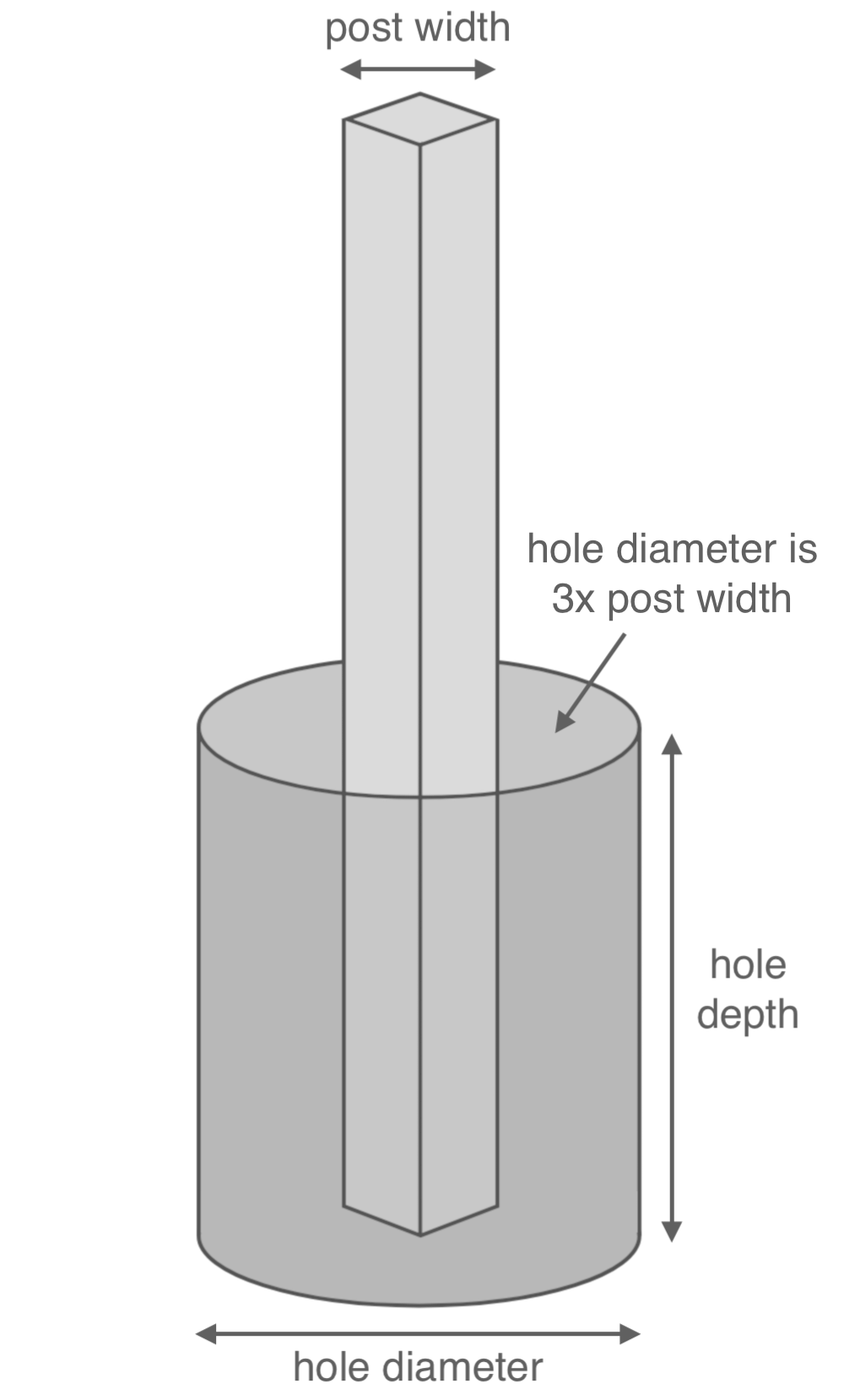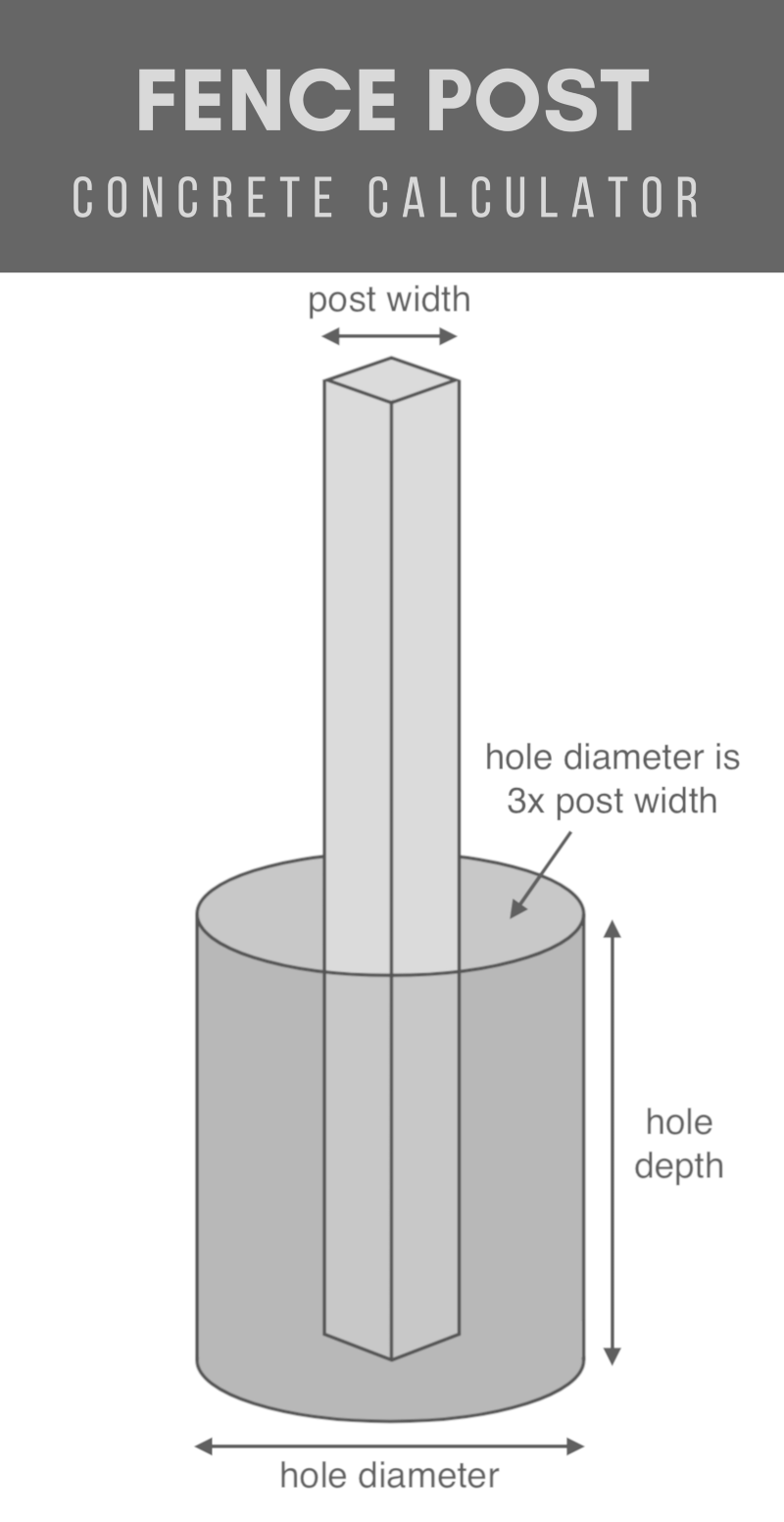# Post Hole Concrete Calculator

Calculate how many yards or how many bags of concrete you’ll need for a set of post holes by entering the post diameter and hole size.

If you’re not sure what size hole to dig, a rule of thumb is to use a hole diameter three times the post diameter and 13 as deep as the post height.

Post Dimensions
Hole Dimensions
The diameter of the hole should be three times the diameter of the post
Optionally enter the price per cubic yard
Average is \$115 per yd3, although your price will vary based on the type of concrete, vendor, and location.
\$
per yd3

## Results:

### Estimated Cost

\$
Learn how we calculated this below

## Using the Post Hole Calculator

Use the post hole estimator to quickly find out how much concrete you will need to set your fence posts. Enter your post and hole dimensions in any units.

The calculator will give you the results in cubic yards and the number of pre-mixed bags of concrete (40 lb, 60 lb, and 80 lb bags).

To ensure that your posts are sturdy for a long time to come, remember to make your holes three times the diameter of your post and 13 as deep as your fence. So, a six-foot high fence needs holes a minimum of two feet deep.

Order additional material to account for settling and spillage. If you have many posts to set, it may pay you to mix the concrete yourself. Or, if you need a cubic yard or more, order mixed and delivered.

Concrete contractors can provide estimates for delivering and installing cement for your post project.Illustration showing a post hole with a diameter that is three times the post’s diameter and with a depth that is 1/3 the height of the post.

## How Much Concrete Do You Need Per Fence Post?

Concrete is sold pre-made (and usually delivered) by the cubic yard or in pre-mixed bags by weight.

To calculate how much concrete you need, you need to know the post size you are going to use and how many posts you are installing. Your post diameter and height determine how big each hole needs to be.

As stated above, fence post holes should be three times the diameter of your post and 13 as deep as your post.

First, you need to calculate the volume for the post hole. Then calculate the volume for the part of the post that will be submerged in the hole. Make sure all your units are the same!

To easily use the table above, convert your units to feet if they aren’t already. Use our calculators to quickly convert inches, yards, or meters to feet.

### Post Hole Volume Formulas

Use these formulas to calculate volume or, for round holes, use our cylinder cubic yardage calculator.

Rectangle:
volume = length × width × depth

Cylinder:
volume = radius2 × π × depth (π = 3.14)

### Calculate Concrete Volume

With the volume of the submerged post and the volume of the hole figured out, subtract the post volume from the hole volume, and you will have the volume of concrete needed for each hole.

Simply multiply that amount times the number of posts to get a total quantity. Don’t forget to add in some extra for spillage and waste.

If you have multiple different size post holes to fill, calculate each set with matching dimensions separately, then add the totals together.

### Example Fence Post Concrete Calculation

Let’s use an example to illustrate what we covered above. Let’s assume you’re installing a 6-foot high fence, where 2 feet of the post will be in the ground, and you will need twenty (20) 4″ nominal round posts.

Note that 4″ posts are actually 3 ½ in diameter. Use the formulas above for volume.

#### Calculate the post volume

Divide by 12 to convert the post diameter to feet:

3.5″ ÷ 12 = 0.2917′

Next, find radius (½ of the diameter):

0.2917′ ÷ 2 = 0.1458′

Finally, use the cylinder formula to find the volume of round post:

(0.1458′)² × π × 2′ = 0.1336 cubic ft.

#### Calculate the hole volume

3 x 0.1458′ = 0.4374′

Find the volume of post hole using the cylinder volume formula:

(0.4374′)² × π × 2′ = 1.2026 cubic ft.

#### Calculate concrete volume

To find the concrete needed, start by subtracting the volume of the post from the volume of the hole:

1.2026 – 0.1336 = 1.069 cubic ft. per post

Then multiply by the number of posts to find the total amount of concrete needed for the project:

1.069 cubic ft × 20 posts = 21.38 cubic ft.

Finally, convert it to cubic yards:

21.38 cubic ft. ÷ 27 = 0.79 cubic yards

Hint: you can also use a concrete calculator for this step.

Most people find it easier to find cubic feet first, then convert it to cubic yards; if so, divide the cubic footage by 27.

## How Many Bags of Concrete Do You Need Per Fence Post

Most concrete suppliers will not sell fractions of a yard of concrete, though it is worth asking about. So, in this example, you would want to buy bags.

Using the table below, you would need to buy at least eight 80 lb. bags and one 40 lb. bag (yielding a total of 5.1 cubic feet) of concrete to allow enough for extra.### Pre-Mix Bag Concrete Yield

The following table shows the approximate yield of mixed concrete for common bag weights.

Approximate yield for various bags of concrete
Bag Concrete Weight Mixed Concrete Yield (approximate)
40 lb (18.1 kg) 0.30 cu ft (8.5 L)
60 lb (27.2 kg) 0.45 cu ft (12.7 L)
80 lb (36.3 kg) 0.60 cu ft (17 L)

## Tips For Ordering Concrete

Consider these tips to ensure your concrete installation goes smoothly.

### Type of Concrete and its Proper Installation

This depends on the application and the climate where you live. Colder temperatures need longer curing times; sandy soils need different shaped holes; more extreme freeze/thaw cycles need higher strength concrete than more temperate regions.

Your concrete supplier can advise you on the proper mix to use for your project and region.

For ready-mix concrete delivery, there are typically additional fees for delivery, weekend service, small loads, and overtime for long unloading times. Discuss these issues with your concrete supplier ahead of time so that you have a clear understanding of costs and can plan accordingly.